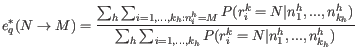### Updating equations for the error rates

For the updating equations for the error probabilities, we consider a read,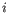, at a given site,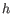. The joint probability of the true nucleotide in the read,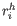, at the site being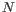and the data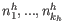is: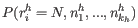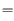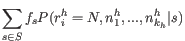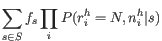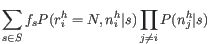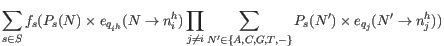(28.7)

Using Bayes formula again, as we did above in 28.4, we get: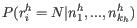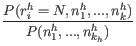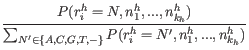and inserting the expression from equation 28.7: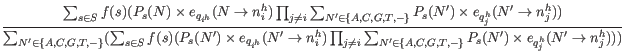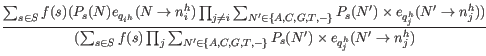(28.8)

The equation 28.9 gives us the probabilities for a given read,, and site,, given the data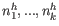, that the true nucleotide is,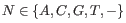, given our current values of the error rates and site probabilities. Since we know the sequenced nucleotide in each read at each site, we can get new updated values for the error rate of producing an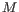nucleotide when the true nucleotide is,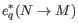, for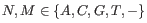by summing the probabilities of the true nucleotide beingfor all reads across all sites for which the sequenced nucleotide is, and dividing by the sum of all probabilities of the true nucleotide being aacross all reads and all sites: